# 《联邦学习实战》：从零开始通过联邦学习实现图像分类

## 《联邦学习实战》：从零开始通过联邦学习实现图像分类

### 一、环境配置

1. 使用Anaconda来配置环境，先将Anaconda更新到最新版本

2. 进入Anaconda命令窗口，产看配置的环境：`conda env list`

3. 发现之前只装了tensorflow的环境，没有pytorch的环境，所以创建一个，检查python版本为3.8，命名为mypytorch1，命令如下：

`conda create -n mypytorch1 python=3.8`4. 激活环境，进入该环境

`conda activate mypytorch1`

5. 根据需要的pytorch去官网赋值命令语句下载，慢的话去找镜像

6. 下完之后查看是否已经安装

`conda list`

7. tips：也可以在 Anaconda Navigator中查看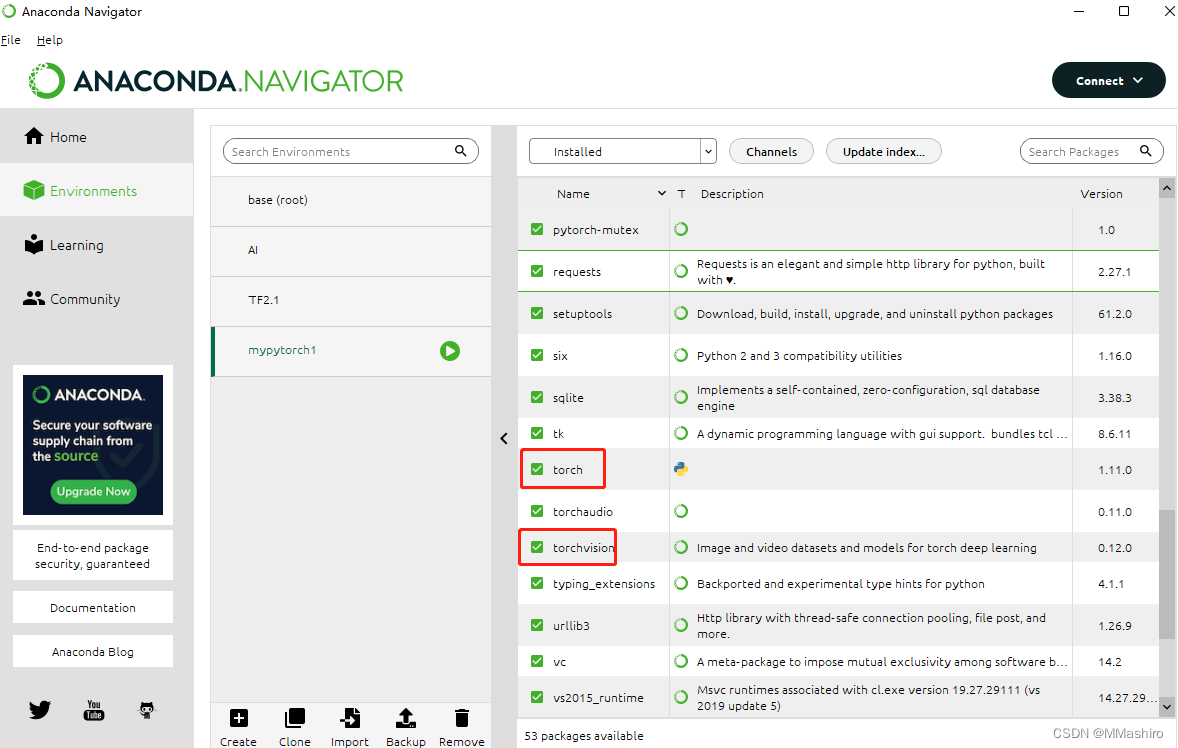8. 检查环境是否配置成果，出现True说明成功

9. `python`
10. `import torch`
11. `print(torch.cuda.is_available())`
12. Pycharm设置，在File→setting中去选择刚才配置好的环境，配好后如图所示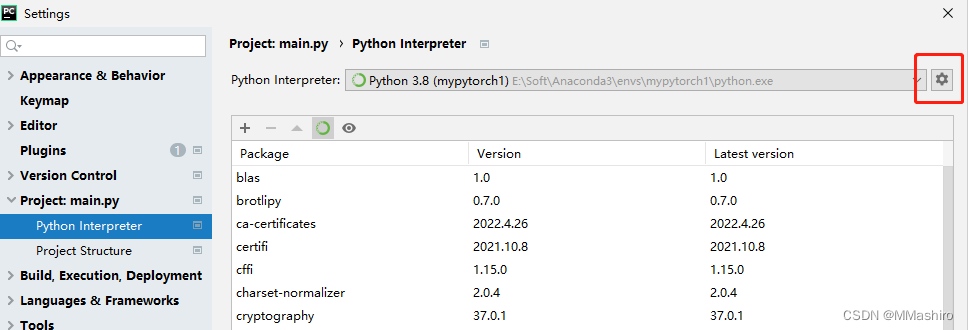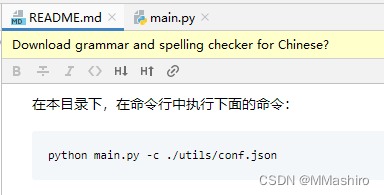`python main.py -c ./utils/conf.json`

14. 一开始还是报错了，找了半天原因没想到重启Pycharm就OK了，期间还用jupyter跑了一遍。

15. 不管怎么说，先跑起来为先。

### 二、运行结果

1. 在jupyter运行结果如下：pycharm运行结果：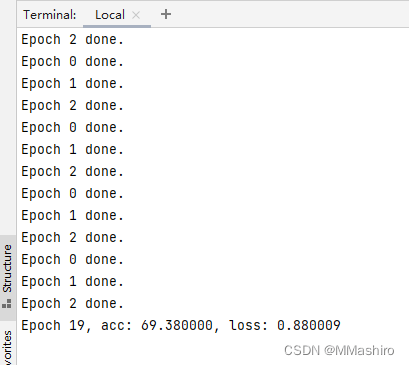### 四、代码分析-Python实现横向联邦图像分类

#### 4.1 总体结构

• 目的：用横向联邦来实现对cifar10图像数据集的分类
• 模型：ResNet-18
• 角色：服务端、客户端和配置文件
• 注意：为了方便实现，本章没有采用网络通信的方式来模拟客户端和服务端的通信，而是在本地以循环的方式来模拟
• #### 4.2 配置信息

联邦学习在模型训练之前，会将配置信息分别发送到服务端和客户端中保存，如果配置信息发生改变，也会同时对所有参与方进行同步，以保证各参与方的配置信息一致。

配置文件`conf.json`信息如下，为便于理解，添加了注释

``````{
//模型信息：即当前任务使用的模型结构，此处为ResNet-18图像分类模型
"model_name" : "resnet18",

//训练的客户端数量：每一轮的迭代，服务端会首先从所有的客户端中挑选部分客户端进行本地训练
//每一次迭代只选取部分客户端参与，并不会影响全局收敛的效果，且能够提升训练的效率
"no_models" : 10,

//数据信息：联邦学习训练的数据，此处使用cifar10数据集
//为了模拟横向建模，数据集将按样本维度，切分为多份不重叠的数据
//每一份放置在每一个客户端中作为本地训练数据
"type" : "cifar",

//全局迭代次数：即服务端和客户端的通信次数
//通常会设置一个最大的全局迭代次数，但在训练过程中，只要模型满足收敛的条件，那么训练也可以提前终止
"global_epochs" : 20,

//本地模型的迭代次数：即每一个客户端在进行本地模型训练时的迭代次数
"local_epochs" : 3,

//每次选取k个客户端参与迭代
"k" : 5,

//本地模型进行训练时的参数-每个batch的大小
"batch_size" : 32,

//本地模型进行训练时的参数-学习率
"lr" : 0.001,

//本地模型进行训练时的参数-momentum
"momentum" : 0.0001,

//本地模型进行训练时的参数-正则化参数
"lambda" : 0.1
}
``````

#### 4.3 训练数据集

按照上述配置文件中的type字段信息，获取数据集，这里用的是torchvision的datasets模块内置的cifar10数据集

注：CIFAR-10 是由 Hinton 的学生 Alex Krizhevsky 和 Ilya Sutskever 整理的一个用于识别普适物体的小型数据集。一共包含 10 个类别的 RGB 彩色图 片：飞机（ a叩lane ）、汽车（ automobile ）、鸟类（ bird ）、猫（ cat ）、鹿（ deer ）、狗（ dog ）、蛙类（ frog ）、马（ horse ）、船（ ship ）和卡车（ truck ）。图片的尺寸为 32×32 ，数据集中一共有 50000 张训练圄片和 10000 张测试图片。

`datasets.py`代码如下

``````import torch
from torchvision import datasets, transforms

def get_dataset(dir, name):
if name=='mnist':
eval_dataset = datasets.MNIST(dir, train=False, transform=transforms.ToTensor())

elif name=='cifar':
# transform：图像类型的转换
# 用Compose串联多个transform操作
transform_train = transforms.Compose([
# 四周填充0，图像随机裁剪成32*32
# 图像一半概率翻转，一半概率不翻转
transforms.RandomHorizontalFlip(),
# 将图片(Image)转成Tensor，归一化至[0, 1]
transforms.ToTensor(),
# 标准化至[-1, 1]，规定均值和标准差
transforms.Normalize((0.4914, 0.4822, 0.4465), (0.2023, 0.1994, 0.2010)),
])

transform_test = transforms.Compose([
# 将图片(Image)转成Tensor，归一化至[0, 1]
transforms.ToTensor(),
# 标准化至[-1, 1]，规定均值和标准差
transforms.Normalize((0.4914, 0.4822, 0.4465), (0.2023, 0.1994, 0.2010)),
])

#得到训练集
transform=transform_train)
#得到测试集
eval_dataset = datasets.CIFAR10(dir, train=False, transform=transform_test)

# 该函数返回训练集和测试集
return train_dataset, eval_dataset
``````

#### 4.4 服务端

`server.py` 代码

注：横向联邦学习的服务端的主要功能是将被选择的客户端上传的本地模型进行模型聚合。但这里需要特别注意的是，事实上，对于一个功能完善的联邦学习框架，比如FATE平台，服务端的功能要复杂得多，比如服务端需要对各个客户端节点进行网络监控、对失败节点发出重连信号等。本章由于是在本地模拟的，不涉及网络通信细节和失败故障等处理，因此不讨论这些功能细节，仅涉及模型聚合功能

下面定义一个服务端类Server，类中的主要函数包括以下三种：

• 定义构造函数

1. 将配置信息拷贝到服务端中
2. 按照配置中的模型信息获取模型，这里使用的是torchvision的models模块内置的ResNet-18模型
3. 模型下载后，令其作为全局初始模型
``````class Server(object):

# 服务端初始化所需参数：配置信息和测试集
def __init__(self, conf, eval_dataset):
# 将配置信息拷贝到服务端中
self.conf = conf
# 按照配置中的模型信息获取模型，这里使用的是torchvision的models模块内置的ResNet-18模型
# 模型下载后，令其作为全局初始模型
self.global_model = models.get_model(self.conf["model_name"])
# 生成一个测试集合加载器
# shuffle=True打乱数据集
``````
• 定义模型聚合函数

1. 在类中定义模型聚合函数，通过接收客户端上传的模型，使用聚合函数更新全局模型
2. 聚合方案有很多种，这里采用经典的FedAvg算法，书中提供的公式如下
3.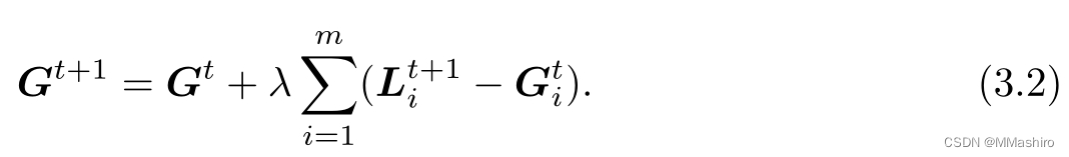``````	# 全局聚合模型
def model_aggregate(self, weight_accumulator):
# 遍历服务器的全局模型
for name, data in self.global_model.state_dict().items():
# weight_accumulator存储了每个客户端上传的参数变化值
# 更新每一层乘上学习率
update_per_layer = weight_accumulator[name] * self.conf["lambda"]
# 累加和
if data.type() != update_per_layer.type():
# update_per_layer的type如果是floatTensor，则将其转换为模型的LongTensor（有一定的精度损失）
else:
``````
• 定义模型评估函数

​ 对当前的全局模型，利用评估数据评估当前的全局模型性能。通常情况下，服务端的评估函数主要对当前聚合后的全局模型进行分析，用于判断当前的模型训练是需要进行下一轮迭代、还是提前终止，或者模型是否出现发散退化的现象。根据不同的结果，服务端可以采取不同的措施策略。

``````	def model_eval(self):
# 开启模型评估模式（不修改参数）
self.global_model.eval()

total_loss = 0.0
correct = 0
dataset_size = 0
#enumerate()函数用于将一个可遍历的数据对象(如列表、元组或字符串)组合为一个索引序列,同时列出数据和数据下标,一般用在for循环当中
data, target = batch
# 获取所有的样本总量大小
dataset_size += data.size()
# 存储到gpu
if torch.cuda.is_available():
data = data.cuda()
target = target.cuda()
# 加载到模型中训练
output = self.global_model(data)

# 把损失值聚合起来
# cross_entropy函数是pytorch中计算交叉熵的函数
# cross_entropy交叉熵函数计算损失
total_loss += torch.nn.functional.cross_entropy(output, target,
reduction='sum').item()
# 获取最大的对数概率的索引值，在所有预测结果中选择可能性最大的作为最终的分类结果
pred = output.data.max(1)
#.item()的作用主要是把数据从tensor取出来，变成python的数据类型，方便后续处理
# 经过view_as()操作后，target.data转变为了与pred相同的形状
# 统计预测结果与真实标签target的匹配总个数
correct += pred.eq(target.data.view_as(pred)).cpu().sum().item()
# 计算准确率
acc = 100.0 * (float(correct) / float(dataset_size))
# 计算损失值
total_l = total_loss / dataset_size

return acc, total_l
``````
• #### 4.5 客户端

`client.py` 代码

横向联邦学习的客户端主要功能是接收服务端的下发指令和全局模型，并利用本地数据进行局部模型训练

本节仅考虑客户端本地的模型训练细节, 首先定义客户端类Client，类中的主要函数包括以下两种

• 定义构造函数

1. 将配置信息拷贝到客户端中
2. 按照配置中的模型信息获取模型，通常由服务端将模型参数传递给客户端，客户端将该全局模型覆盖掉本地模型
3. 配置本地训练数据，此处通过torchvision的datasets模块获取cifar10数据集后按客户端ID进行切分，不同的客户端拥有不同的子数据集，相互之间没有交集
``````import models, torch, copy
class Client(object):

def __init__(self, conf, model, train_dataset, id = -1):
# 引入配置文件
self.conf = conf
# 客户端本地模型
self.local_model = models.get_model(self.conf["model_name"])
# 客户端ID
self.client_id = id
#客户端本地数据集
self.train_dataset = train_dataset

# 列表(List)是最常用的Python数据类型，它可以作为一个方括号内的逗号分隔值出现
# range(start,stop[,step])
# start:计数从start开始，默认是从0开始。例如range(5)等价于range(0,5);
# all_range是训练集的索引
all_range = list(range(len(self.train_dataset)))
# data_len是每个客户端的数据量
data_len = int(len(self.train_dataset) / self.conf['no_models'])
# 根据客户端的id来平均划分训练集，train_indices为该id下的子训练集
train_indices = all_range[id * data_len: (id + 1) * data_len]

# 训练数据集的加载器，自动将数据分割成batch
# sampler定义从数据集中提取样本的策略
# 使用sampler：构造数据集的SubsetRandomSampler采样器，它会对下标进行采样
# self.train_dataset父集合
# sampler指定子集合
``````
• 定义模型本地训练函数

1. 此处为图像分类的例子，使用交叉熵作为本地模型的损失函数
2. 利用梯度下降来求解并更新参数值
``````	def local_train(self, model):
# 整体的过程：拉取服务器的模型，通过部分本地数据集训练得到
for name, param in model.state_dict().items():
# 客户端首先用服务器端下发的全局模型覆盖本地模型
self.local_model.state_dict()[name].copy_(param.clone())

#print(id(model))
# 定义最优化函数器，用于本地模型训练
optimizer = torch.optim.SGD(self.local_model.parameters(), lr=self.conf['lr'],
momentum=self.conf['momentum'])
#print(id(self.local_model))
# 本地模型训练
self.local_model.train()
for e in range(self.conf["local_epochs"]):

data, target = batch
# 加载到gpu
if torch.cuda.is_available():
data = data.cuda()
target = target.cuda()
# 梯度
# 训练预测
output = self.local_model(data)
# 计算损失函数 cross_entropy交叉熵误差
loss = torch.nn.functional.cross_entropy(output, target)
# 反向传播
loss.backward()
# 更新参数
optimizer.step()
print("Epoch %d done." % e)
# 创建差值字典（结构与模型参数同规格），用于记录差值
diff = dict()
for name, data in self.local_model.state_dict().items():
# 计算训练后与训练前的差值
diff[name] = (data - model.state_dict()[name])
#print(diff[name])

return diff
``````

#### 4.6 整合

`main.py` 代码

1. 当配置文件、服务端类和客户端类都定义完毕后，我们将这些信息组合起来

``````	# 设置命令行程序
parser = argparse.ArgumentParser(description='Federated Learning')
# 获取所有的参数
args = parser.parse_args()

# 读取配置文件
with open(args.conf, 'r') as f:
``````
2. 分别定义一个服务端对象和多个客户端对象，用来模拟横向联邦训练场景

``````	train_datasets, eval_datasets = datasets.get_dataset("./data/", conf["type"])

server = Server(conf, eval_datasets)
clients = []
# 创建多个客户端
for c in range(conf["no_models"]):
clients.append(Client(conf, server.global_model, train_datasets, c))
``````
3. 每一轮的迭代，服务端会从当前的客户端集合中随机挑选一部分参与本轮迭代训练，被选中的客户端调用本地训练接口local_train进行本地训练，最后服务端调用模型聚合函数model_aggregate来更新全局模型

``````	# 全局模型训练
for e in range(conf["global_epochs"]):
# 每次训练都是从clients列表中选取k个客户端参与本次联邦训练
candidates = random.sample(clients, conf["k"])

weight_accumulator = {}

# 初始化权重/初始化空模型参数weight_accumulator
for name, params in server.global_model.state_dict().items():
# 生成一个和参数矩阵大小相同的0矩阵
weight_accumulator[name] = torch.zeros_like(params)

# 遍历客户端，每个客户端本地训练模型
for c in candidates:
diff = c.local_train(server.global_model)

# 根据客户端的参数差值字典更新总体权重
for name, params in server.global_model.state_dict().items():

# 模型参数聚合
server.model_aggregate(weight_accumulator)

# 模型聚合完毕后，调用模型评估接口来评估每一轮更新后的全局模型效果
acc, loss = server.model_eval()

print("Epoch %d, acc: %f, loss: %f\n" % (e, acc, loss))
``````

### 五、对比实验

为了绘制对比曲线，本来想用tensorboard工具，但pytorch用起来好像不是很方便，所以用最笨的方法，将想要对比的情况各自的loss和acc保存起来，然后新建个python文件来对比

保存的过程参考了文章：pytorch训练过程中Loss的保存与读取、绘制Loss图

联邦学习时，参数为上文的参数，在中心化训练时将 no_modelsk 都设置为 1，再调整 local_epochs 运行程序

下面复现实验效果图：

1. 先在`main.py`中增加保存loss和acc值到文件中的语句(此处为中心化训练时保存的语句，联邦学习类似)

``````	Loss_save = np.array(loss_list)
np.save('./result/NON_FL_local_epochs_{}_loss'.format(conf["local_epochs"]), Loss_save)

Acc_save = np.array(acc_list)
np.save('./result/NON_FL_local_epochs_{}_acc'.format(conf["local_epochs"]), Acc_save)
``````
2. 编写新的 `compare.py` 程序来画图

``````import numpy as np
from matplotlib import pyplot as plt

# 中文乱码解决方法
plt.rcParams['font.family'] = ['Arial Unicode MS','Microsoft YaHei','SimHei','sans-serif']
plt.rcParams['axes.unicode_minus'] = False

#联邦训练结果

#中心化训练结果1 local_epochs = 1

#中心化训练结果2 local_epochs = 2

#中心化训练结果3 local_epochs = 3

# 横坐标
# 绘制 loss 曲线
plt.figure(1)
plt.title('Loss Curve')  # 图片标题
plt.xlabel('Epoch')  # x轴变量名称
plt.ylabel('Loss')  # y轴变量名称
plt.plot(epoch_list, loss_load1, 'bp-', label=u"联邦学习(局部迭代三次)")  # 逐点画出loss_list值并连线，连线图标是Loss
plt.plot(epoch_list, loss_load2, 'ro-', label=u"中心化训练(局部迭代一次)")  # 逐点画出loss_list值并连线，连线图标是Loss
plt.plot(epoch_list, loss_load3, 'g+-', label=u"中心化训练(局部迭代两次)")  # 逐点画出loss_list值并连线，连线图标是Loss
plt.plot(epoch_list, loss_load4, 'mx-', label=u"中心化训练(局部迭代三次)")  # 逐点画出loss_list值并连线，连线图标是Loss
plt.xticks(epoch_list)
plt.legend()  # 画出曲线图标
plt.show()  # 画出图像

# 绘制 Accuracy 曲线
plt.figure(2)
plt.title('Acc Curve')  # 图片标题
plt.xlabel('Epoch')  # x轴变量名称
plt.ylabel('Acc')  # y轴变量名称
plt.plot(epoch_list, acc_load1, 'bp-', label=u"联邦学习(局部迭代三次)")  # 逐点画出acc_list值并连线，连线图标是Accuracy
plt.plot(epoch_list, acc_load2, 'ro-', label=u"中心化训练(局部迭代一次)")  # 逐点画出acc_list值并连线，连线图标是Accuracy
plt.plot(epoch_list, acc_load3, 'g+-', label=u"中心化训练(局部迭代两次)")  # 逐点画出acc_list值并连线，连线图标是Accuracy
plt.plot(epoch_list, acc_load4, 'mx-', label=u"中心化训练(局部迭代三次)")  # 逐点画出acc_list值并连线，连线图标是Accuracy
plt.xticks(epoch_list)
plt.legend()
plt.show()
``````
3. 我的运行结果如图所示